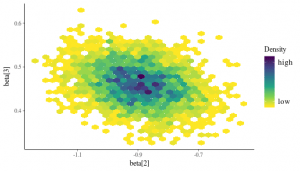# MCMC Using STAN – Diagnostics With The Bayesplot Package: Exercises

April 8, 2018
By

Want to share your content on R-bloggers? click here if you have a blog, or here if you don't.This exercise set will continue to present the STAN platform, but with another useful tool: the `bayesplot` package. This package is very useful to construct diagnostics that can be used to have insights on the convergence of the MCMC sampling since the convergence of the generated chains is the main issue in most STAN models.
You can find the first bit of information on `bayesplot `package in this introductory vignette or in this vignette.

We will make use of a survey of residents from a small area in Bangladesh that was affected by arsenic in drinking water. Respondents with elevated arsenic levels in their wells were asked if they were interested in getting water from a neighbor’s well. A series of logistic regressions could be fit to predict this binary response given various information about the households. Here we fit a model for the well-switching response, given two predictors: the arsenic level of the water in the resident’s home and the distance of the house from the nearest safe well.
More information on this data can be found in this interesting paper.

Solutions for this set of exercises can be found here. The first chunk defines, installs (if needed) and loads the packages needed in this set.

Exercise 1
After having installed and loaded the `rstan `and `bayesplot `packages, set the global options that allows you to automatically save a bare version of a compiled Stan program. This way, it does not need to be recompiled to execute multiple chains, in parallel, by taking all the available cores of your machine (use the `parallel` package).
Set the color “bright blue” so that it applies to all subsequent plots.
Finally, import the data of interest; the wells.dat data-set, you can import from the Gelman repository by simply making use of the `read.table()` function from the R utils package. Create the variable “dist100″ which is equal to `dist/100 `.

Exercise 2
Create a plot to visualize the relationship between the two variables of interest with the dependent binary variable “switch“.
Then, fit the model with the frequentist `glm()` function and inspect the summary of the estimation results.

Exercise 3
Write the STAN model of interest in your R script by following the pertaining blocks of a STAN code by using weak priors for the parameters (ex. take multivariate normal prior with a standard deviation of 10).

The data block that will gather all the data is used in the STAN model: N (the number of data points; it cannot be negative), P (the number of different predictors; it cannot be negative), X (the matrix of covariates, including the intercept) and y (the binary outcome).
The parameters block: beta that is represented as a parameter vector for the parameters of the logistic regression model.
The model block: it defines the prior distribution for the parameters beta and creates the response vector of interest by multiplying the matrix of covariates with the parameters.

Do not forget to constraint the upper and lower bounds in the data and parameter block declarations.

Note that it is recommended to write the STAN code in a separate file (“.stan” extension) and then call it directly through the `stan()` function instead of writing it in a character, like what is done here. That would be easier to debug, read, etc.

Exercise 4
Define the named data list that will be used inside the STAN data block written above.

Exercise 5
Run the STAN model using the `stan()` function and using the following input parameters:
– The STAN code defined in Exercise 3
– The data list defined in Exercise 4
– Four different chains
– 1000 iterations per chain
– A warm-up phase of 200. This phase defines the number of iterations that are used by the sampler for the adaptation phase before sampling begins (and is thus different of the burn-in phase we have seen in the Metropolis or Gibbs samplers).
Use all the available cores of your machine and make it reproducible (take the `seed` 1234). Then, print the estimation outputs of the parameters.

Exercise 6
Plot the central posterior uncertainty intervals of each parameter in the form of horizontal lines with the median as a central point.

Exercise 7
Do the same as Exercise 6, but with the posterior estimates represented by density areas. Take the mean as a central point and 80%/99% as inner/outer credible intervals.

Exercise 8
– Plot the histograms of the three parameters. Take the binwidth of 1/50 for each parameter. Then, do the same, but displaying each of the 4 chains and take a binwidth of 1/100.
– Do the same but with density plots. Overlay chains in the density plots instead of plotting them separately, like the histograms.
– Draw violin-plots of the 4 chains separately for each parameter except the intercept. Highlight the quantiles 5%, 20%, 50%, 70%, and 95%.

Exercise 9
Compute the traceplots for each parameter separately and overlay the 4 pertaining chains in each plot.

Exercise 10
Since we are interested only in the two covariates (dist100 and arsenic), represent a bivariate scatter-plot of the posterior draws. Then, try to avoid over-plotting with hexagonal binning.
Finally, represent a scatter-plot table of all the parameters with the density plots lying in the diagonal and the posterior draws in the off-diagonal.

R-bloggers.com offers daily e-mail updates about R news and tutorials about learning R and many other topics. Click here if you're looking to post or find an R/data-science job.
Want to share your content on R-bloggers? click here if you have a blog, or here if you don't.